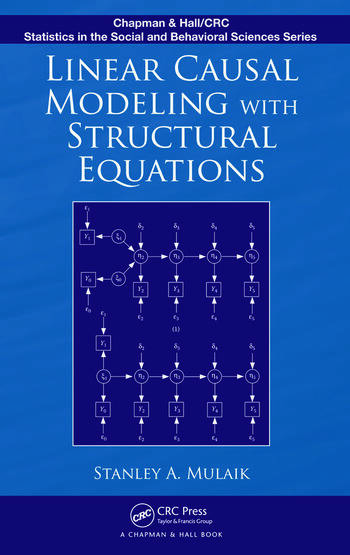# Linear Causal Modeling with Structural Equations

## 1st Edition

Stanley A. Mulaik

Chapman and Hall/CRC
Published June 16, 2009
Reference - 468 Pages - 96 B/W Illustrations
ISBN 9781439800386 - CAT# K10039
Series: Chapman & Hall/CRC Statistics in the Social and Behavioral Sciences

USD\$105.00

FREE Standard Shipping!

## Preview

### Summary

Emphasizing causation as a functional relationship between variables that describe objects, Linear Causal Modeling with Structural Equations integrates a general philosophical theory of causation with structural equation modeling (SEM) that concerns the special case of linear causal relations. In addition to describing how the functional relation concept may be generalized to treat probabilistic causation, the book reviews historical treatments of causation and explores recent developments in experimental psychology on studies of the perception of causation. It looks at how to perceive causal relations directly by perceiving quantities in magnitudes and motions of causes that are conserved in the effects of causal exchanges.

The author surveys the basic concepts of graph theory useful in the formulation of structural models. Focusing on SEM, he shows how to write a set of structural equations corresponding to the path diagram, describes two ways of computing variances and covariances of variables in a structural equation model, and introduces matrix equations for the general structural equation model. The text then discusses the problem of identifying a model, parameter estimation, issues involved in designing structural equation models, the application of confirmatory factor analysis, equivalent models, the use of instrumental variables to resolve issues of causal direction and mediated causation, longitudinal modeling, and nonrecursive models with loops. It also evaluates models on several dimensions and examines the polychoric and polyserial correlation coefficients and their derivation.

Covering the fundamentals of algebra and the history of causality, this book provides a solid understanding of causation, linear causal modeling, and SEM. It takes readers through the process of identifying, estimating, analyzing, and evaluating a range of models.